# RS Aggarwal Solutions for Class 8 Maths Chapter 6 - Operations on Algebraic Expressions Exercise 6D

Learners can download the RS Aggarwal Solutions for the Exercise 6D of Class 8 Maths Chapter 6, Operations on Algebraic Expressions from the link available here. BYJU’S expert team has solved the questions in such a way that learners can understand easily and comfortably. This exercise is based on some special identities which are necessary for higher studies. By practising the RS Aggarwal Solutions for class 8, students will be able to grasp the concepts perfectly.

## Download PDF of RS Aggarwal Solutions for Class 8 Maths Chapter 6 – Operations on Algebraic Expressions Exercise 6D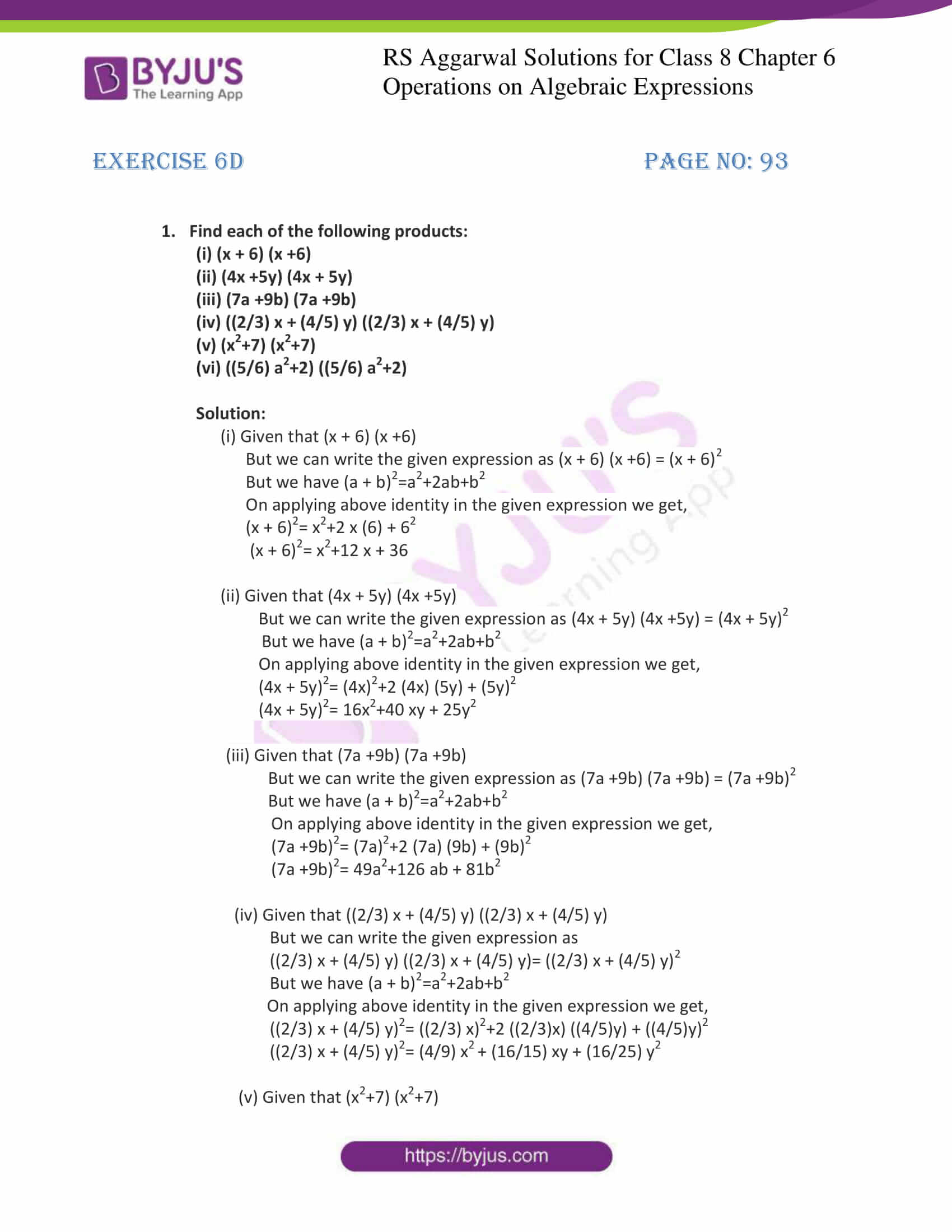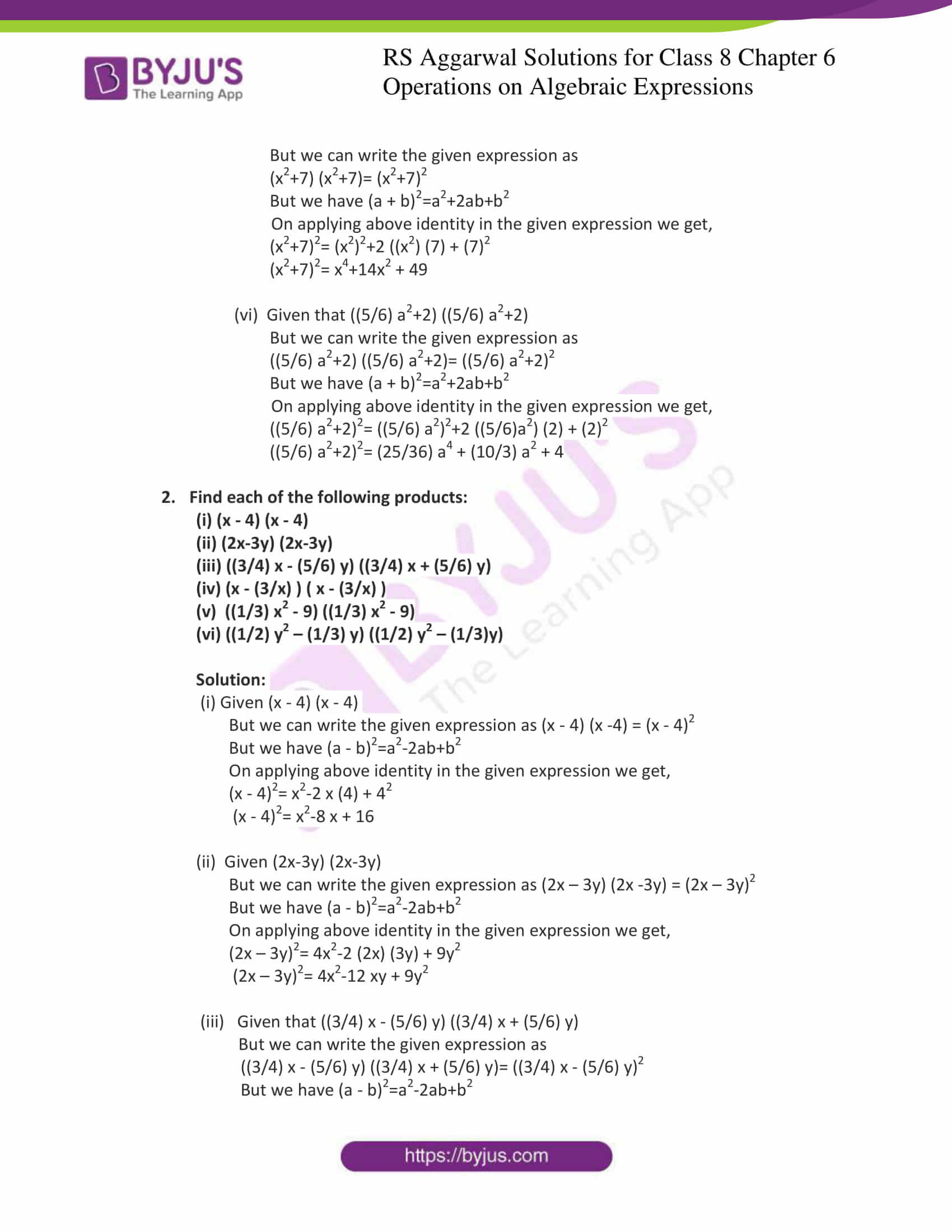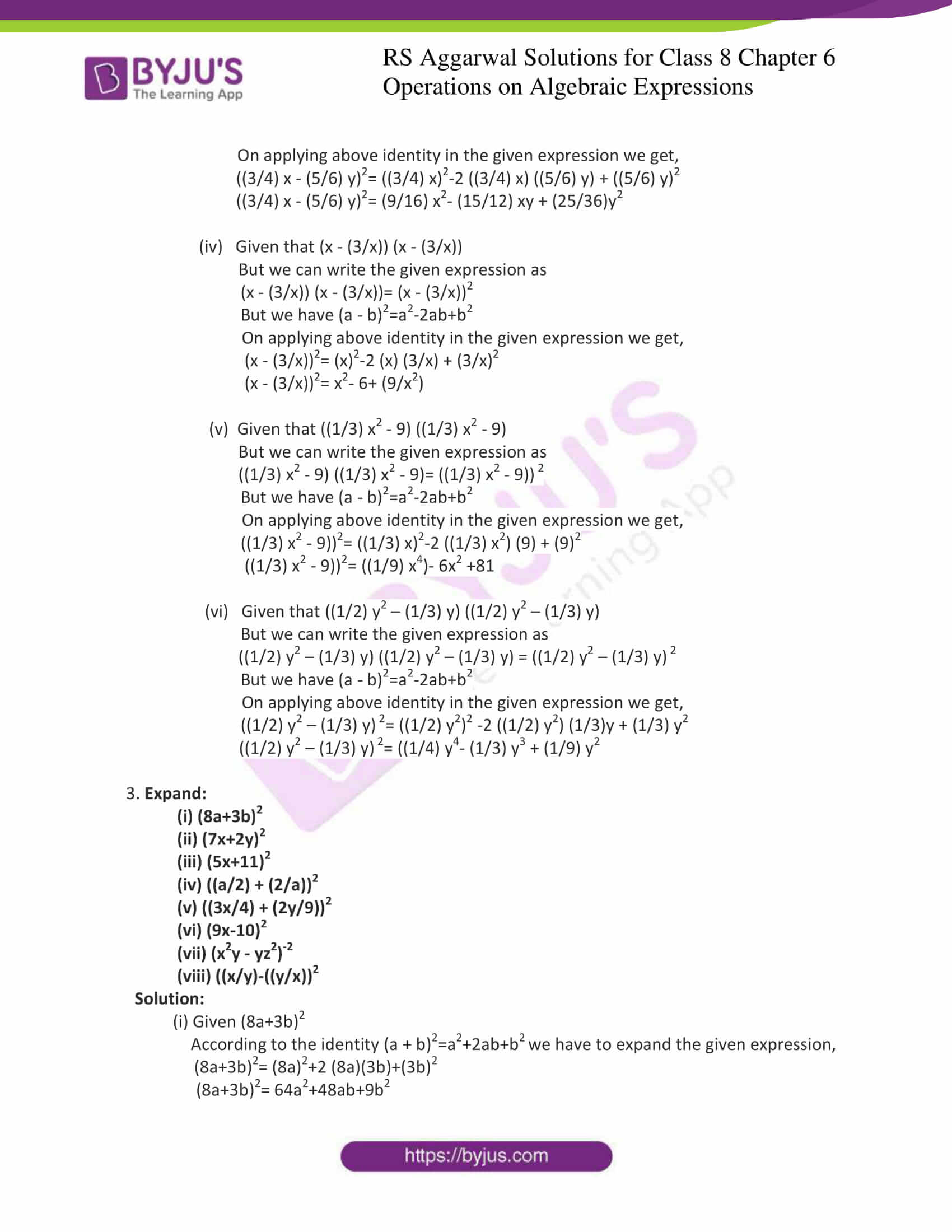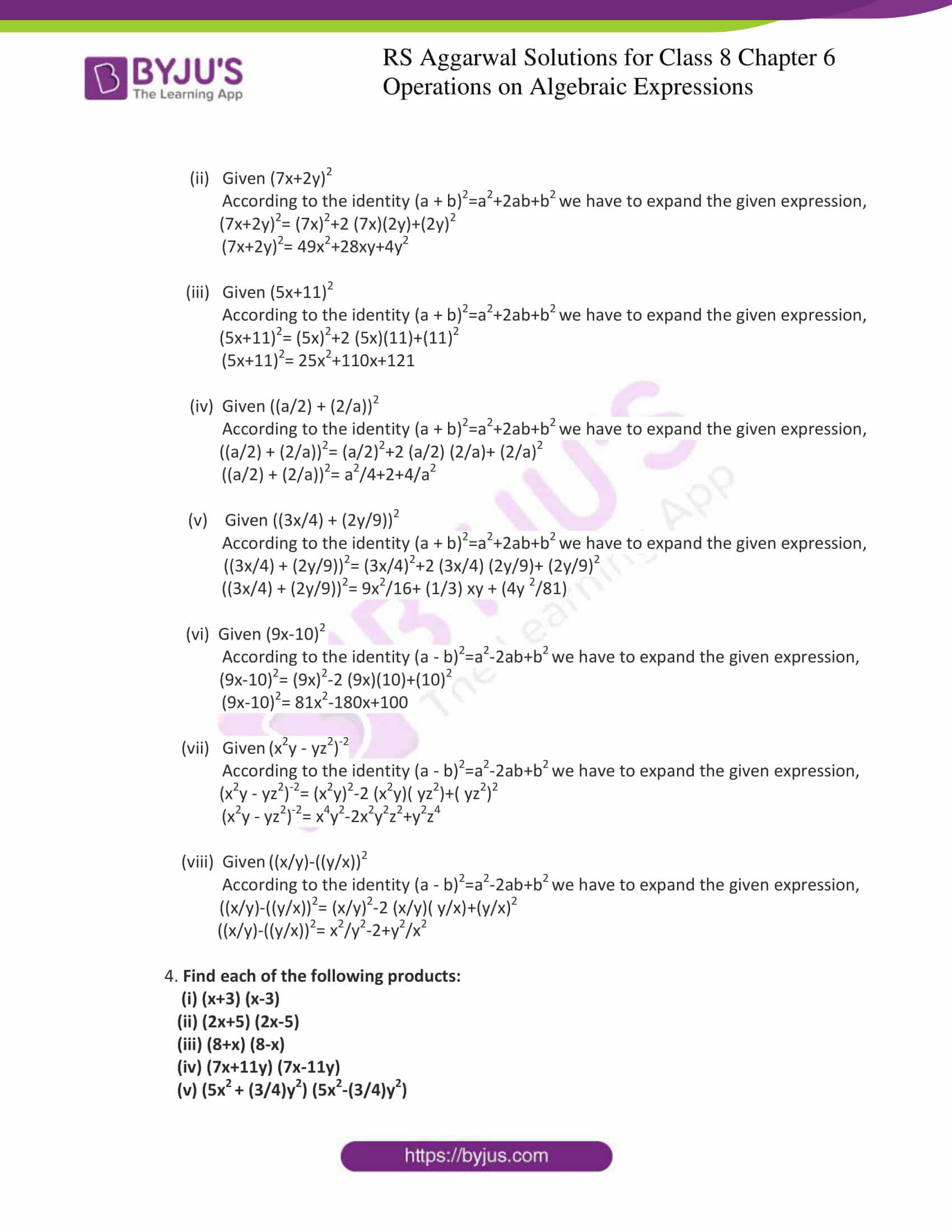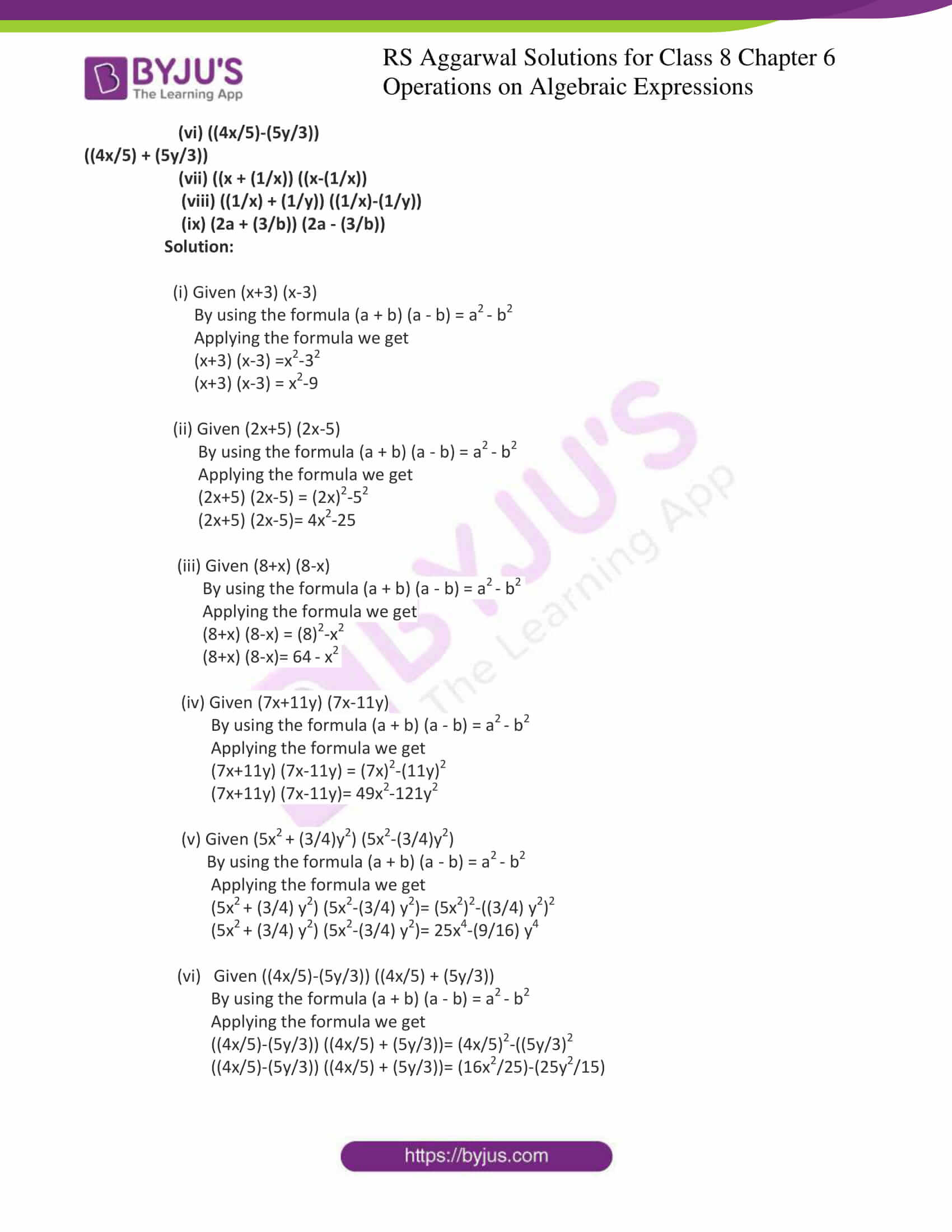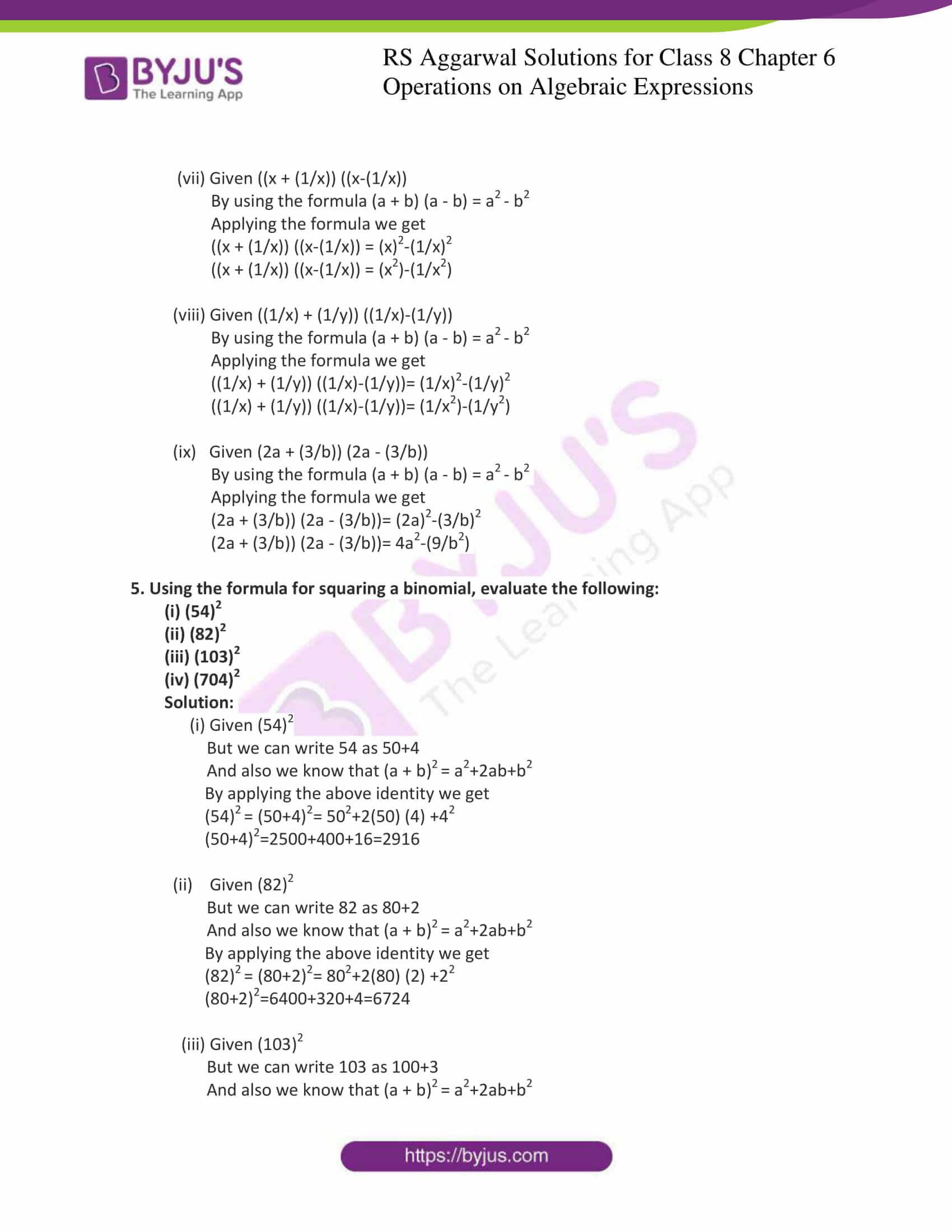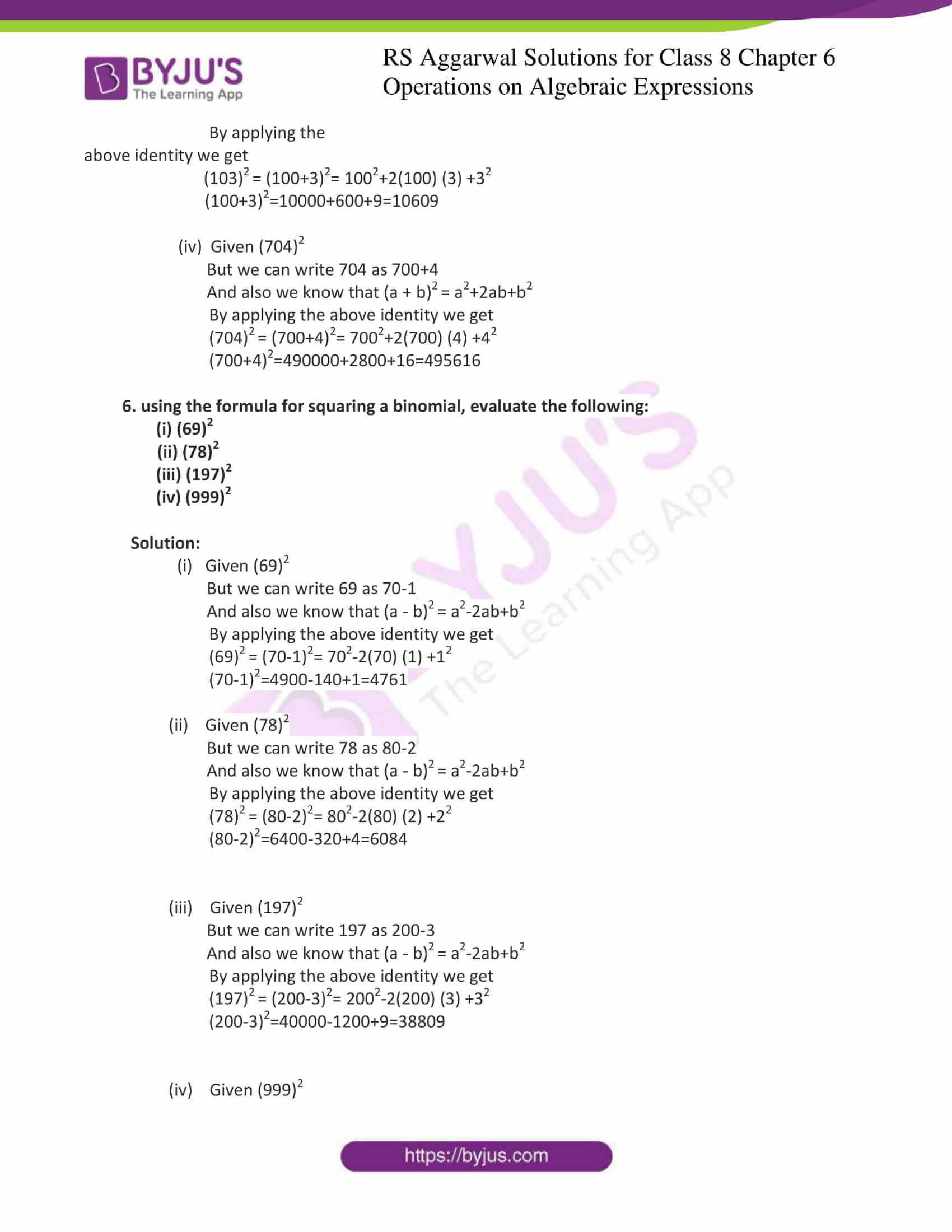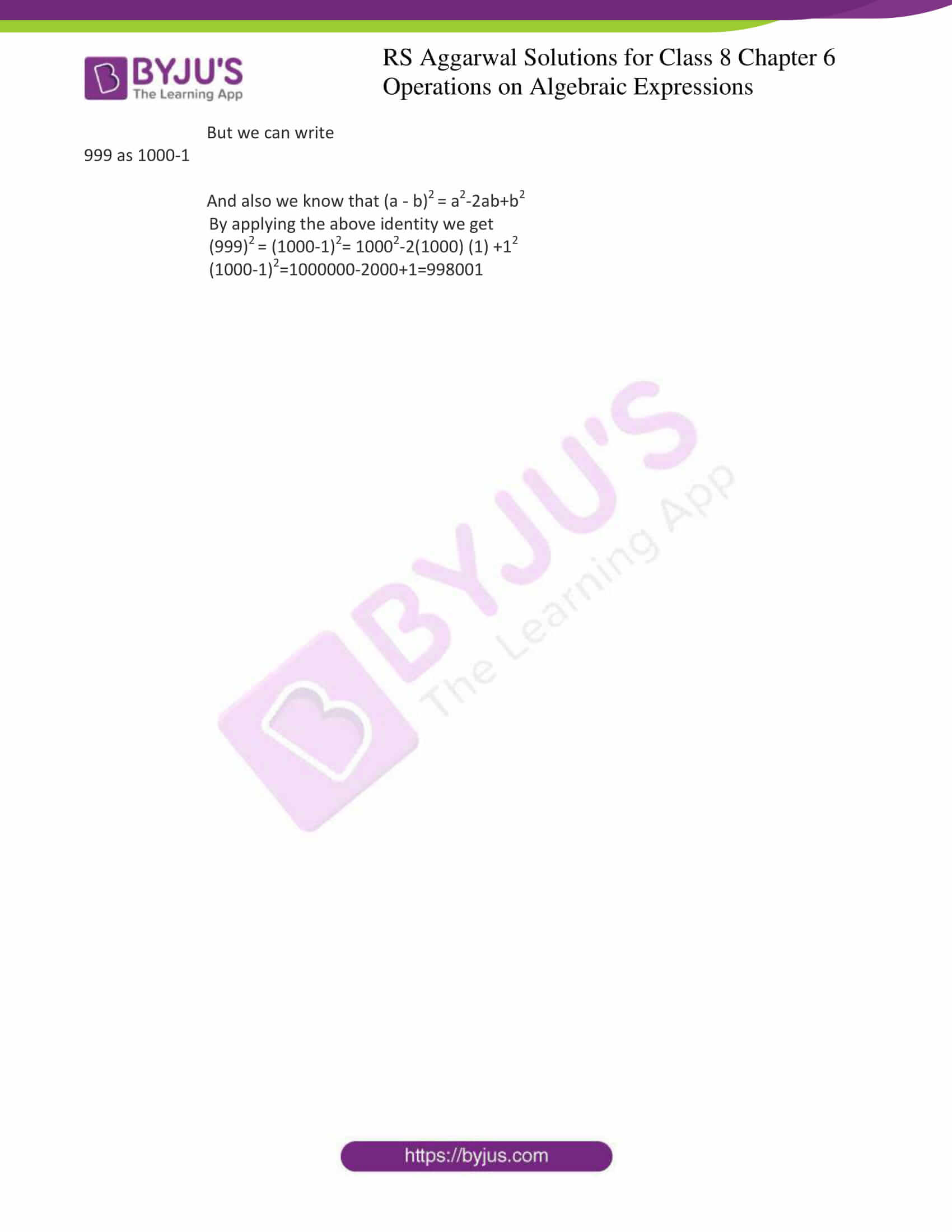### Access answers to Maths RS Aggarwal Solutions for Class 8 Chapter 6 – Operations on Algebraic Expressions Exercise 6D

1. Find each of the following products:

(i) (x + 6) (x +6)

(ii) (4x +5y) (4x + 5y)

(iii) (7a +9b) (7a +9b)

(iv) ((2/3) x + (4/5) y) ((2/3) x + (4/5) y)

(v) (x2+7) (x2+7)

(vi) ((5/6) a2+2) ((5/6) a2+2)

Solution:

(i) Given that (x + 6) (x +6)

But we can write the given expression as (x + 6) (x +6) = (x + 6)2

But we have (a + b)2=a2+2ab+b2

On applying above identity in the given expression we get,

(x + 6)2= x2+2 x (6) + 62

(x + 6)2= x2+12 x + 36

(ii) Given that (4x + 5y) (4x +5y)

But we can write the given expression as (4x + 5y) (4x +5y) = (4x + 5y)2

But we have (a + b)2=a2+2ab+b2

On applying above identity in the given expression we get,

(4x + 5y)2= (4x)2+2 (4x) (5y) + (5y)2

(4x + 5y)2= 16x2+40 xy + 25y2

(iii) Given that (7a +9b) (7a +9b)

But we can write the given expression as (7a +9b) (7a +9b) = (7a +9b)2

But we have (a + b)2=a2+2ab+b2

On applying above identity in the given expression we get,

(7a +9b)2= (7a)2+2 (7a) (9b) + (9b)2

(7a +9b)2= 49a2+126 ab + 81b2

(iv) Given that ((2/3) x + (4/5) y) ((2/3) x + (4/5) y)

But we can write the given expression as

((2/3) x + (4/5) y) ((2/3) x + (4/5) y)= ((2/3) x + (4/5) y)2

But we have (a + b)2=a2+2ab+b2

On applying above identity in the given expression we get,

((2/3) x + (4/5) y)2= ((2/3) x)2+2 ((2/3)x) ((4/5)y) + ((4/5)y)2

((2/3) x + (4/5) y)2= (4/9) x2 + (16/15) xy + (16/25) y2

(v) Given that (x2+7) (x2+7)

But we can write the given expression as

(x2+7) (x2+7)= (x2+7)2

But we have (a + b)2=a2+2ab+b2

On applying above identity in the given expression we get,

(x2+7)2= (x2)2+2 ((x2) (7) + (7)2

(x2+7)2= x4+14x2 + 49

(vi) Given that ((5/6) a2+2) ((5/6) a2+2)

But we can write the given expression as

((5/6) a2+2) ((5/6) a2+2)= ((5/6) a2+2)2

But we have (a + b)2=a2+2ab+b2

On applying above identity in the given expression we get,

((5/6) a2+2)2= ((5/6)a2 )2+2 ((5/6)a2 ) (2) + (2)2

((5/6) a2+2)2= (25/36) a4+ (10/3) a2 + 4

2. Find each of the following products:

(i) (x – 4) (x – 4)

(ii) (2x-3y) (2x-3y)

(iii) ((3/4) x – (5/6) y) ((3/4) x + (5/6) y)

(iv) (x – (3/x) ) ( x – (3/x) )

(v) ((1/3) x2 – 9) ((1/3) x2 – 9)

(vi) ((1/2) y2 – (1/3) y) ((1/2) y2 – (1/3)y)

Solution:

(i) Given (x – 4) (x – 4)

But we can write the given expression as (x – 4) (x -4) = (x – 4)2

But we have (a – b)2=a2-2ab+b2

On applying above identity in the given expression we get,

(x – 4)2= x2-2 x (4) + 42

(x – 4)2= x2-8 x + 16

(ii) Given (2x-3y) (2x-3y)

But we can write the given expression as (2x – 3y) (2x -3y) = (2x – 3y)2

But we have (a – b)2=a2-2ab+b2

On applying above identity in the given expression we get,

(2x – 3y)2= 4x2-2 (2x) (3y) + 9y2

(2x – 3y)2= 4x2-12 xy + 9y2

(iii) Given that ((3/4) x – (5/6) y) ((3/4) x + (5/6) y)

But we can write the given expression as

((3/4) x – (5/6) y) ((3/4) x + (5/6) y)= ((3/4) x – (5/6) y)2

But we have (a – b)2=a2-2ab+b2

On applying above identity in the given expression we get,

((3/4) x – (5/6) y)2= ((3/4) x)2-2 ((3/4) x) ((5/6) y) + ((5/6) y)2

((3/4) x – (5/6) y)2= (9/16) x2– (15/12) xy + (25/36)y2

(iv) Given that (x – (3/x)) (x – (3/x))

But we can write the given expression as

(x – (3/x)) (x – (3/x))= (x – (3/x))2

But we have (a – b)2=a2-2ab+b2

On applying above identity in the given expression we get,

(x – (3/x))2= (x)2-2 (x) (3/x) + (3/x)2

(x – (3/x))2= x2– 6+ (9/x2)

(v) Given that ((1/3) x2 – 9) ((1/3) x2 – 9)

But we can write the given expression as

((1/3) x2 – 9) ((1/3) x2 – 9)= ((1/3) x2 – 9)) 2

But we have (a – b)2=a2-2ab+b2

On applying above identity in the given expression we get,

((1/3) x2 – 9))2= ((1/3) x)2-2 ((1/3) x2 ) (9) + (9)2

((1/3) x2 – 9))2= ((1/9) x4)- 6x2 +81

(vi) Given that ((1/2) y2 – (1/3) y) ((1/2) y2 – (1/3) y)

But we can write the given expression as

((1/2) y2 – (1/3) y) ((1/2) y2 – (1/3) y) = ((1/2) y2 – (1/3) y) 2

But we have (a – b)2=a2-2ab+b2

On applying above identity in the given expression we get,

((1/2) y2 – (1/3) y) 2= ((1/2) y2)2 -2 ((1/2) y2 ) (1/3) + (1/3) y2

((1/2) y2 – (1/3) y) 2= ((1/4) y2– y3 (1/3) + (1/9) y2

3. Expand:

(i) (8a+3b)2

(ii) (7x+2y)2

(iii) (5x+11)2

(iv) ((a/2) + (2/a))2

(v) ((3x/4) + (2y/9))2

(vi) (9x-10)2

(vii) (x2y – yz2)-2

(viii) ((x/y)-((y/x))2

Solution:

(i) Given (8a+3b)2

According to the identity (a + b)2=a2+2ab+b2 we have to expand the given expression,

(8a+3b)2= (8a)2+2 (8a)(3b)+(3b)2

(8a+3b)2= 64a2+48ab+9b2

(ii) Given (7x+2y)2

According to the identity (a + b)2=a2+2ab+b2 we have to expand the given expression,

(7x+2y)2= (7x)2+2 (7x)(2y)+(2y)2

(7x+2y)2= 49x2+28xy+4y2

(iii) Given (5x+11)2

According to the identity (a + b)2=a2+2ab+b2 we have to expand the given expression,

(5x+11)2= (5x)2+2 (5x)(11)+(11)2

(5x+11)2= 25x2+110x+121

(iv) Given ((a/2) + (2/a))2

According to the identity (a + b)2=a2+2ab+b2 we have to expand the given expression,

((a/2) + (2/a))2= (a/2)2+2 (a/2) (2/a)+ (2/a)2

((a/2) + (2/a))2= a2/4+2+4/a2

(v) Given ((3x/4) + (2y/9))2

According to the identity (a + b)2=a2+2ab+b2 we have to expand the given expression,

((3x/4) + (2y/9))2= (3x/4)2+2 (3x/4) (2y/9)+ (2y/9)2

((3x/4) + (2y/9))2= 9x2/16+ (1/3) xy + (4y 2/81)

(vi) Given (9x-10)2

According to the identity (a – b)2=a2-2ab+b2 we have to expand the given expression,

(9x-10)2= (9x)2-2 (9x)(10)+(10)2

(9x-10)2= 81x2-180x+100

(vii) Given (x2y – yz2)-2

According to the identity (a – b)2=a2-2ab+b2 we have to expand the given expression,

(x2y – yz2)-2= (x2y)2-2 (x2y)( yz2)+( yz2)2

(x2y – yz2)-2= x4y2-2x2y2z2+y2z4

(viii) Given ((x/y)-((y/x))2

According to the identity (a – b)2=a2-2ab+b2 we have to expand the given expression,

((x/y)-((y/x))2= (x/y)2-2 (x/y)( y/x)+(y/x)2

((x/y)-((y/x))2= x2/y2-2+y2/x2

4. Find each of the following products:

(i) (x+3) (x-3)

(ii) (2x+5) (2x-5)

(iii) (8+x) (8-x)

(iv) (7x+11y) (7x-11y)

(v) (5x2 + (3/4)y2) (5x2-(3/4)y2)

(vi) ((4x/5)-(5y/3)) ((4x/5) + (5y/3))

(vii) ((x + (1/x)) ((x-(1/x))

(viii) ((1/x) + (1/y)) ((1/x)-(1/y))

(ix) (2a + (3/b)) (2a – (3/b))

Solution:

(i) Given (x+3) (x-3)

By using the formula (a + b) (a – b) = a2 – b2

Applying the formula we get

(x+3) (x-3) =x2-32

(x+3) (x-3) = x2-9

(ii) Given (2x+5) (2x-5)

By using the formula (a + b) (a – b) = a2 – b2

Applying the formula we get

(2x+5) (2x-5) = (2x)2-52

(2x+5) (2x-5)= 4x2-25

(iii) Given (8+x) (8-x)

By using the formula (a + b) (a – b) = a2 – b2

Applying the formula we get

(8+x) (8-x) = (8)2-x2

(8+x) (8-x)= 64 – x2

(iv) Given (7x+11y) (7x-11y)

By using the formula (a + b) (a – b) = a2 – b2

Applying the formula we get

(7x+11y) (7x-11y) = (7x)2-(11y)2

(7x+11y) (7x-11y)= 49x2-121y2

(v) Given (5x2 + (3/4)y2) (5x2-(3/4)y2)

By using the formula (a + b) (a – b) = a2 – b2

Applying the formula we get

(5x2 + (3/4) y2) (5x2-(3/4) y2)= (5x2)2-((3/4) y2)2

(5x2 + (3/4) y2) (5x2-(3/4) y2)= 25x4-(9/16) y4

(vi) Given ((4x/5)-(5y/3)) ((4x/5) + (5y/3))

By using the formula (a + b) (a – b) = a2 – b2

Applying the formula we get

((4x/5)-(5y/3)) ((4x/5) + (5y/3))= (4x/5)2-((5y/3)2

((4x/5)-(5y/3)) ((4x/5) + (5y/3))= (16x2/25)-(25y2/15)

(vii) Given ((x + (1/x)) ((x-(1/x))

By using the formula (a + b) (a – b) = a2 – b2

Applying the formula we get

((x + (1/x)) ((x-(1/x)) = (x)2-(1/x)2

((x + (1/x)) ((x-(1/x)) = (x2)-(1/x2)

(viii) Given ((1/x) + (1/y)) ((1/x)-(1/y))

By using the formula (a + b) (a – b) = a2 – b2

Applying the formula we get

((1/x) + (1/y)) ((1/x)-(1/y))= (1/x)2-(1/y)2

((1/x) + (1/y)) ((1/x)-(1/y))= (1/x2)-(1/y2)

(ix) Given (2a + (3/b)) (2a – (3/b))

By using the formula (a + b) (a – b) = a2 – b2

Applying the formula we get

(2a + (3/b)) (2a – (3/b))= (2a)2-(3/b)2

(2a + (3/b)) (2a – (3/b))= 4a2-9/b2

5. Using the formula for squaring a binomial, evaluate the following:

(i) (54)2

(ii) (82)2

(iii) (103)2

(iv) (704)2

Solution:

(i) Given (54)2

But we can write 54 as 50+4

And also we know that (a + b)2 = a2+2ab+b2

By applying the above identity we get

(54)2 = (50+4)2= 502+2(50) (4) +42

(50+4)2=2500+400+16=2916

(ii) Given (82)2

But we can write 82 as 80+2

And also we know that (a + b)2 = a2+2ab+b2

By applying the above identity we get

(82)2 = (80+2)2= 802+2(80) (2) +22

(80+2)2=6400+320+4=6724

(iii) Given (103)2

But we can write 103 as 100+3

And also we know that (a + b)2 = a2+2ab+b2

By applying the above identity we get

(103)2 = (100+3)2= 1002+2(100) (3) +32

(100+3)2=10000+600+9=10609

(iv) Given (704)2

But we can write 704 as 700+4

And also we know that (a + b)2 = a2+2ab+b2

By applying the above identity we get

(704)2 = (700+4)2= 7002+2(700) (4) +42

(700+4)2=490000+2800+16=495616

6. using the formula for squaring a binomial, evaluate the following:

(i) (69)2

(ii) (78)2

(iii) (197)2

(iv) (999)2

Solution:

(i) Given (69)2

But we can write 69 as 70-1

And also we know that (a – b)2 = a2-2ab+b2

By applying the above identity we get

(69)2 = (70-1)2= 702-2(70) (1) +12

(70-1)2=4900-140+1=4761

(ii) Given (78)2

But we can write 78 as 80-2

And also we know that (a – b)2 = a2-2ab+b2

By applying the above identity we get

(78)2 = (80-2)2= 802-2(80) (2) +22

(80-2)2=6400-320+4=6084

(iii) Given (197)2

But we can write 197 as 200-3

And also we know that (a – b)2 = a2-2ab+b2

By applying the above identity we get

(197)2 = (200-3)2= 2002-2(200) (3) +32

(200-3)2=40000-1200+9=38809

(iv) Given (999)2

But we can write 999 as 1000-1

And also we know that (a – b)2 = a2-2ab+b2

By applying the above identity we get

(999)2 = (1000-1)2= 10002-2(1000) (1) +12

(1000-1)2=1000000-2000+1=998001

Exercise 6A

Exercise 6B

Exercise 6C

Exercise 6E

## RS Aggarwal Solutions for Class 8 Maths Chapter 6 – Operations on Algebraic Expressions Exercise 6D

Exercise 6D of RS Aggarwal Solutions for Chapter 6, Operations on Algebraic Expressions deals with the basic concepts related to special identities of Algebraic Expressions. Some of the topics focused prior to exercise 6D include the following.

• Identities
• Applications of identities

The RS Aggarwal Solutions can help the students in practising and learning each and every concept as it provides solutions to all questions asked in the RS Aggarwal textbook. Those who aim to score high in the Maths of Class 8 are advised to practice all the questions present in the RS Aggarwal as many times as possible.